# Cluster algorithms

Cluster algorithms are mainly used in the simulation of Ising-like models using Monte Carlo methods. The essential feature is the use of collective motions of particles (spins) in a single Monte Carlo step. An interesting property of some of these applications is the fact that the percolation analysis of the clusters can be used to study phase transitions.

## Swendsen-Wang algorithm

As an introductory example to the Swendsen-Wang algorithm we shall discuss the technique  in the simulation of the Ising model. In one Monte Carlo step of the algorithm the following recipe is used:

1. Consider every pair of interacting sites (spins). In the current configuration the pair interaction can be either negative: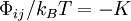$\Phi_{ij}/k_B T= -K$ or positive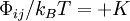$\Phi_{ij}/k_B T = + K$, depending on the product: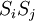$S_{i} S_{j}$ (See the Ising model entry for notation).
2. For pairs of interacting sites (i.e. nearest neighbours) with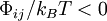$\Phi_{ij}/k_B T < 0$, randomly create a bond between the two spins with a given probability$p$, where$p$ will be chosen to be a function of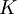$K$.
3. The bonds generated in the previous step are used to build up clusters of sites (spins).
4. Build up the partition of the system in the corresponding clusters of spins. In each cluster all the spins will have the same state, either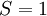$S = 1$ or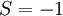$S = -1$.
5. For each cluster, independently, choose at random with equal probabilities whether or not to flip (invert the value of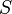$S$) the whole set of spins belonging to the cluster. The bonding probability$p$ is given by: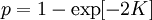$p = 1 - \exp [ -2 K ]$.

## Wolff algorithm

The procedure to create a given bond is the same as in the Swendsen-Wang algorithm. However in Wolff's method the whole set of interacting pairs is not tested to generate (possible) bonds. Instead, a single cluster is built. See  for details.

1. The initial cluster contains one site, which is selected at random.
2. Possible bonds between the initial site and other sites of the system are tested. Bonded sites are included in the cluster.
3. Recursively, one checks the existence of bonds between the new members of the cluster and sites of the system to add, if bonds are generated, new sites to the growing cluster, until no more bonds are generated.
4. At this point, the whole cluster is flipped (see above).

The purpose of this algorithm is to locate critical points (i.e. the critical temperature). So, in this case the probability of bonding neighbouring sites with equal spins is not set a priori (See ). The algorithm for an Ising system with periodic boundary conditions can be implemented as follows:

Given a certain configuration of the system:

1. One considers the possible bonds in the system (pairs of nearest neighbours with favourable interaction).
2. One assigns a random order to these possible bonds.
3. The possible bonds are activated in the order fixed in the previous step (the cluster structure is watched during this process).
4. The bond activation stops when one cluster percolates through the entire system (i.e. considering the periodic boundary conditions the cluster becomes of infinite size).
5. Every cluster is then is flipped with probability 1/2, as in the Swendsen-Wang algorithm.
6. An effective bond probability for the percolation threshold,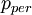$p_{per}$ can be computed as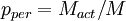$p_{per} = M_{act}/M$ with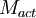$M_{act}$ being the number of activated bonds when the first cluster percolates, and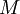$M$ is the number of possible bonds.
7. The value of$p_{per}$ (in one realisation, or the averaged value over the simulation, see references for a practical application) can be related with the critical coupling constant,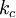$k_c$ as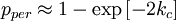$p_{per} \approx 1 - \exp \left[ - 2 k_c \right]$.

## Probability-Changing Cluster Algorithm

This method was proposed by Tomita and Okabe . This procedure is orientated towards computing critical points. It applies when the symmetry of the interactions imply that the critical temperature is that in which the clusters, built using a Swendsen-Wang type algorithm, reach the percolation threshold. The simulation proceeds by a fine tuning of the temperature (or the coupling constant) Given a configuration of the system and a current coupling constant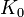$K_0$:

1. One builds a bond realisation following the Swendsen-Wang strategy
2. One establishes whether at least one of the cluster percolates through the whole system
3. If percolation occurs one decreases the coupling constant (increase the temperature) by a small amount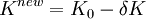$K^{new} = K_0 - \delta K$
4. If no percolation appears, the new value of the coupling constant is taken to be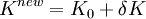$K^{new} = K_0 + \delta K$, with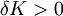$\delta K > 0$.

For small values of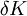$\delta K$ the value of$K$ (after reaching the vicinity of the critical point) will show minor oscillations and the results can be trusted to be those of an equilibrium simulation run. (note that detailed balance is not strictly fulfilled in this algorithm).

## Beyond the Ising and Potts models

The methods described so far can be used, with minor changes, in the simulation of Potts models. In addition, extensions have been proposed in the literature to build up very efficient cluster algorithms to simulate more complex lattice systems (for example the XY model, Heisenberg model, Lebwohl-Lasher model , etc.)

## Application to continuous (atomistic) models

It is sometimes possible (and very convenient) to include cluster algorithms in the simulation of models with continuous translational degrees of freedom. In most cases the cluster algorithm has to be complemented with other sampling moves to ensure ergodicity. Examples:

In these cases, the usual approach is to combine one-particle moves (e.g. particle translations), with cluster procedures. In the cluster steps, multiparticle modification of -composition, orientations, etc.- is carried out.

## Geometric cluster algorithms

Geometric methods have been proposed for the efficient simulation of continuum fluids  , and have also been applied to simulations of mixtures,  such as colloids .

## Other applications of cluster algorithms

The cluster algorithms described so far are rejection-free methods, which means that every new configuration generated throughout the sampling is accepted. However, when the complexity of models increases, it becomes difficult to develop efficient rejection-free algorithms. Nevertheless, in some cases it is still sometimes possible to build up quite efficient cluster algorithms.

Examples:

• Collective (cluster) translation/rotations in the simulation of the primitive model of electrolytes.